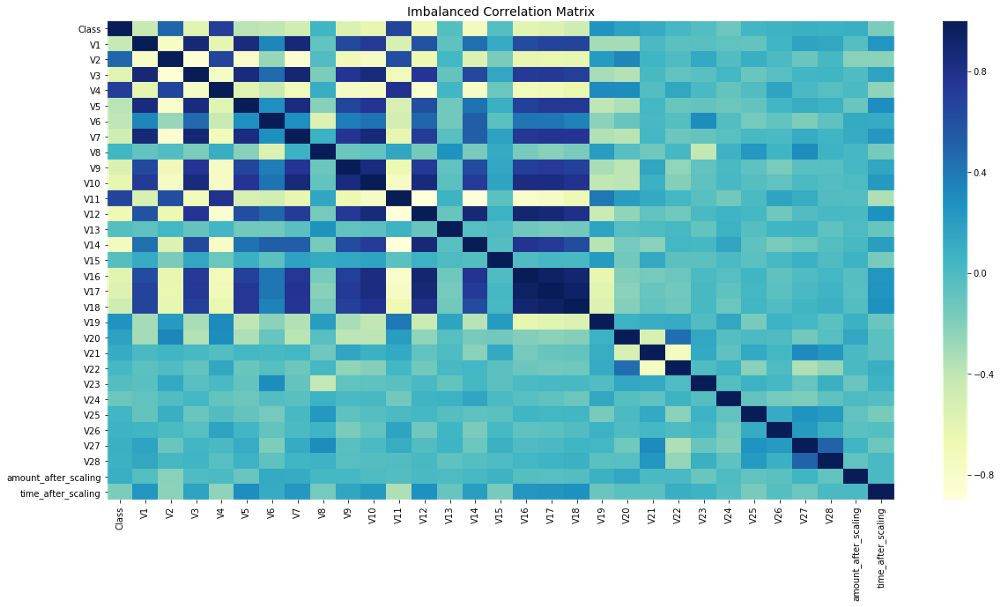Auto ByteScience AI# 一文教你如何处理不平衡数据集（附代码）

#### 什么是数据不平衡（类别不平衡）？

https://www.kaggle.com/mlg-ulb/creditcardfraudhttps://github.com/wmlba/innovate2019/blob/master/Credit_Card_Fraud_Detection.ipynb

#### 一、 重采样（过采样和欠采样）# Shuffle the Dataset.

shuffled_df = credit_df.sample(frac=1,random_state=4)

# Put all the fraud class in a separate dataset.

fraud_df = shuffled_df.loc[shuffled_df['Class'] == 1]

#Randomly select 492 observations from the non-fraud (majority class)

non_fraud_df=shuffled_df.loc[shuffled_df['Class']== 0].sample(n=492,random_state=42)

# Concatenate both dataframes again

normalized_df = pd.concat([fraud_df, non_fraud_df])

#plot the dataset after the undersampling

plt.figure(figsize=(8, 8))

sns.countplot('Class', data=normalized_df)

plt.title('Balanced Classes')

plt.show()imbalanced-learn

from imblearn.over_sampling import SMOTE

# Resample the minority class. You can change the strategy to 'auto' if you are not sure.

sm = SMOTE(sampling_strategy='minority', random_state=7)

# Fit the model to generate the data.

oversampled_trainX,oversampled_trainY=sm.fit_sample(credit_df.drop('Class', axis=1), credit_df['Class'])

oversampled_train=pd.concat([pd.DataFrame(oversampled_trainY), pd.DataFrame(oversampled_trainX)], axis=1)

oversampled_train.columns = normalized_df.columns

# Sample figsize in inches

fig, ax = plt.subplots(figsize=(20,10))

# Imbalanced DataFrame Correlation

corr = credit_df.corr()

sns.heatmap(corr, cmap='YlGnBu', annot_kws={'size':30}, ax=ax)

ax.set_title("Imbalanced Correlation Matrix", fontsize=14)

plt.show()https://towardsdatascience.com/why-feature-correlation-matters-a-lot-847e8ba439c4

#### 二、 集成方法（采样器集成）

BalancedBaggingClassifier

https://mp.weixin.qq.com/cgi-bin/appmsg?t=media/appmsg_edit&action=edit&type=10&isMul=1&isNew=1&lang=zh_CN&token=89565677#imblearn.ensemble.BalancedBaggingClassifier

from imblearn.ensemble import BalancedBaggingClassifier

from sklearn.tree import DecisionTreeClassifier

#Create an object of the classifier.

bbc = BalancedBaggingClassifier(base_estimator=DecisionTreeClassifier(),

sampling_strategy='auto',

replacement=False,

random_state=0)

y_train = credit_df['Class']

X_train = credit_df.drop(['Class'], axis=1, inplace=False)

#Train the classifier.

bbc.fit(X_train, y_train)

preds = bbc.predict(X_train)

How to fix an Unbalanced Dataset

https://www.kdnuggets.com/2019/05/fix-unbalanced-dataset.htmlTHU数据派

THU数据派"基于清华，放眼世界"，以扎实的理工功底闯荡“数据江湖”。发布全球大数据资讯，定期组织线下活动，分享前沿产业动态。了解清华大数据，敬请关注姐妹号“数据派THU”。